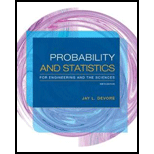# The article “Evaluating Variability in Filling Operations” ( Food Tech ., 1984: 51–55 ) describes two different filling operations used in a ground-beef packing plant. Both filling operations were set to fill packages with 1400 g of ground beef. In a random sample of size 30 taken from each filling operation, the resulting means and standard deviations were 1402.24 g and 10.97 g for operation 1 and 1419.63 g and 9.96 g for operation 2. a. Using a .05 significance level, is there sufficient evidence to indicate that the true mean weight of the packages differs for the two operations? b. Does the data from operation 1 suggest that the true mean weight of packages produced by operation 1 is higher than 1400 g? Use a .05 significance level.BuyFind

### Probability and Statistics for Eng...

9th Edition
Jay L. Devore
Publisher: Cengage Learning
ISBN: 9781305251809BuyFind

### Probability and Statistics for Eng...

9th Edition
Jay L. Devore
Publisher: Cengage Learning
ISBN: 9781305251809

#### Solutions

Chapter 9, Problem 93SE
Textbook Problem

## Expert Solution

### Want to see the full answer?

Check out a sample textbook solution.

### Want to see this answer and more?

Experts are waiting 24/7 to provide step-by-step solutions in as fast as 30 minutes!*

*Response times vary by subject and question complexity. Median response time is 34 minutes and may be longer for new subjects.

#### Additional Math Textbook Solutions

Find more solutions based on key concepts
Explain why the formula for sample variance is different from the formula for population variance.

Essentials of Statistics for The Behavioral Sciences (MindTap Course List)

Expand each expression in Exercises 122. x(4x+6)

Finite Mathematics and Applied Calculus (MindTap Course List)

Find the derivative of the function. F (x) = (1 + x + x2)99

Single Variable Calculus: Early Transcendentals, Volume I

Solve each equation: a. 12x2 9x 3 = 0 b. 3x2 5x + 1 = 0

Applied Calculus for the Managerial, Life, and Social Sciences: A Brief Approach

In Problems 7-34, perform the indicated operations and simplify. 25.

Mathematical Applications for the Management, Life, and Social Sciences

In Exercises 9-14, state whether the statements are true or false. a. Ab. AA

Finite Mathematics for the Managerial, Life, and Social Sciences

The graph at the right has equation:

Study Guide for Stewart's Multivariable Calculus, 8th

is the binomial series for:

Study Guide for Stewart's Single Variable Calculus: Early Transcendentals, 8th

The price per share of stock for a sample of 25 companies was recorded at the beginning of 2012 and then again ...

Modern Business Statistics with Microsoft Office Excel (with XLSTAT Education Edition Printed Access Card) (MindTap Course List)

Reminder Round all answers to two decimal places unless otherwise indicated. Exponential Growth Rate and Genera...

Functions and Change: A Modeling Approach to College Algebra (MindTap Course List)

In Problems 2932 express f in terms of unit step functions. Find f(t) and etf(t). 32. Figure 7.R.9 Graph for Pr...

A First Course in Differential Equations with Modeling Applications (MindTap Course List)© 2021 bartleby. All Rights Reserved.